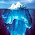### leetcode Question 105: Subsets II

Subsets II
Given a collection of integers that might contain duplicates, S, return all possible subsets.
Note:
• Elements in a subset must be in non-descending order.
• The solution set must not contain duplicate subsets.
For example,
If S = [1,2,2], a solution is:
[
,
,
[1,2,2],
[2,2],
[1,2],
[]
]
Analysis:
Be careful with the question, for [1,2,2], [2,2] is needed.
Here we use another way different from the previous problem to solve this problem.

First, consider there is no duplicates, how to generate the subsets?
Say n is the # of the elements,
when n=1, subsets :  {}, {"1"},  "i" means the ith element.
when n=2, subsets:   {}, {"1"}, {"2"}, {"1", "2"}
when n=3, subsets:   {}, {"1"}, {"2"}, {"1", "2"}, {"3"}, {"1","3"}, {"2","3"}, {"1", "2","3"}
So, the way of generating subsets is:
From 2 to n, COPY the previous subsets, add the current element, push back to the subsets list.

Then we take the duplicates into account, the same example:
when n=1, subsets :  {}, {"1"},  "i" means the ith element.
when n=2, subsets:   {}, {"1"}, {"2"}, {"1", "2"}
when n=3, but "2"=="3" subsets:
{}, {"1"}, {"2"}, {"1", "2"}, {"3"}, {"1","3"}, {"2","3"}, {"1", "2","3"}
since "2"=="3", which truly is:
{}, {"1"}, {"2"}, {"1", "2"}, {"2"}, {"1","2"}, {"2","2"}, {"1", "2","2"}
where the bold ones are not needed.
So, how these two subsets are generated? They are from the subsets of n=1.

In sum up, when meet the same element as previous one, then generate new subsets ONLY from the subsets generated from previous iteration, other than the whole subsets list.

See code below for more details.

Code(Updated 201309):
class Solution {
public:
vector<vector<int> > subsetsWithDup(vector<int> &S) {
// Start typing your C/C++ solution below
// DO NOT write int main() function
sort(S.begin(),S.end());
vector<vector<int> > res;
vector<int> r;
res.push_back(r);
r.push_back(S);
res.push_back(r);
int pre = S;
int count = 1;
for (int i=1;i<S.size();i++){
int st=0;
int sz = res.size();
if (S[i]==pre){st = sz-count;}
count =0;
for (int j=st;j<sz;j++){
r = res[j];
r.push_back(S[i]);
res.push_back(r);
count++;
}
pre=S[i];
}
return res;
}
};


Code:
class Solution {
public:
vector<vector<int> > subsetsWithDup(vector<int> &S) {
// Start typing your C/C++ solution below
// DO NOT write int main() function
sort(S.begin(),S.end());
vector<vector<int> > res;
vector<int> ss;
res.push_back(ss);
ss.push_back(S);
res.push_back(ss);
int count=1;
int pre = S;
for (int i=1;i<S.size();i++){
int sz = res.size();
if (S[i]!=pre){
count=0;
for (int j=0;j<sz;j++){
ss=res[j];
ss.push_back(S[i]);
res.push_back(ss);
count++;
}
}else{
int ind=count;
count=0;
for (int j=sz-ind;j<sz;j++){
ss=res[j];
ss.push_back(S[i]);
res.push_back(ss);
count++;
}

}
pre = S[i];
}
return res;
}
};


#### 1 comment:

1.This comment has been removed by the author.